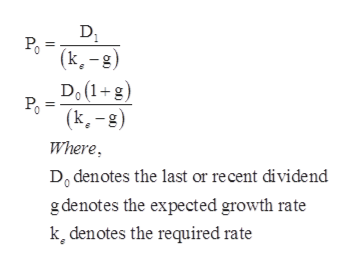# Ferradini company's stock has a required rate of return of 12.50% and it sells for \$18.00 per share. Ferradini's dividend is expected to grow at a constant rate of 7.00%. what was the last dividend?

Question
12 views

Ferradini company's stock has a required rate of return of 12.50% and it sells for \$18.00 per share. Ferradini's dividend is expected to grow at a constant rate of 7.00%. what was the last dividend?

check_circle

Step 1

Formula to calculate the last dividend:help_outlineImage TranscriptioncloseDJ (k-g) Do(1+g) (k-g) Where Do denotes the last or recent dividend g denotes the expected growth rate k, denotes the required rate fullscreen
Step 2

Calculation of last divide...

### Want to see the full answer?

See Solution

#### Want to see this answer and more?

Solutions are written by subject experts who are available 24/7. Questions are typically answered within 1 hour.*

See Solution
*Response times may vary by subject and question.
Tagged in